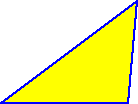The sum of the measures of the interior angles of a triangle is 180 degrees.

Since all the sides of equilateral triangles are the same length, all the angles are the same...The interior angles of an equilateral triangle are all 60 degrees.

What about a square?

That's easy! By definition, all the interior angles of a square are right angles -- That means that they are all 90 degrees.What about other regular polygons?

To figure out the measure of the interior angles of a regular pentagon, hexagon, heptagon, etc, we need more than just a protractor! What if we needed to find the interior angle of a regular polygon with 100 sides? That might be a little difficult to draw!

Here are two methods to find the measure of the interior angles of a regular polygon:

For both methods, we will use the fact that the sum of the measures of the interior angles of a triangle is 180 degrees!